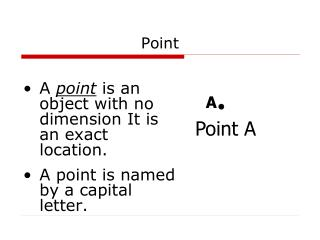DownloadDownload PresentationPoint

# Point

Télécharger la présentation## Point

- - - - - - - - - - - - - - - - - - - - - - - - - - - E N D - - - - - - - - - - - - - - - - - - - - - - - - - - -
##### Presentation Transcript

1. A point is an object with no dimension It is an exact location. A point is named by a capital letter. Point A Point A

2. A line is a straight path that extends without end in one dimension or two opposite directions Line l A B Line l or AB

3. Points are said to be collinear points if they are on the same line Collinear l A B Line l or AB

4. A plane is a flat surface that extends without end in two dimensions A M C B Plane Plane M or plane ABC

5. Points are said to be coplanar if they are on the same plane A M C B Coplanar Plane M or plane ABC

6. The line segment is made up of two endpoints and all the points between the endpoints. Line segment A B Segment AB l B A Line l or AB

7. A ray has one initial point. From that point, the ray extends without end in one direction only. Ray A B Ray AB l B A Line l or AB

8. Two rays are opposite rays if they go in exactly opposite directions, forming a line Opposite Rays l B C A Line l or AB

9. Warm-Up • Name all line segments and rays. A F Z K B

10. Intersection • Geometric figures intersect if they have one or more points in common Line l Line k F E A D B C

11. Rules of Intersection • The intersection of two lines is a point • The intersection of two planes is a line

12. Postulates, Axioms, and Theorems • Postulate or axiom—a rule accepted without proof • Theorem—a statement that can be proven using other rules • Example: Axiom—Two points determine a line

13. Practice

14. Ruler Postulate • Coordinate—a real number value assigned to a point • The distance between A and B is AB = | x2 – x1 | A B x1 x2

15. Segment Addition Postulate • If C is a point between A and B, then AB + BC = AC • If AB + BC = AC, then C is a point between A and B

16. Practice • P. 14 #48-51 • P. 15 #62-67 • P. 21 #23-33

17. Warm-Up Sketch the figure. • 1) A line and a plane that do not intersect. • 2) Two planes that do not intersect and a line that intersects each plane in one point. Simplify. • 3) |4-7| 4) √(4+32)

18. ?s

19. The Coordinate Plane • Recall the definition of coordinate. • The coordinate plane is a grid wherein points are assigned two values

20. Plotting Points • Plot the points • A(3,-5) • B(1,-2) • C(3,0) • D(-4,0)

21. The Distance Formula • If A(x1 , y1) and B (x2 , y2) are points in a coordinate plane, then the distance between them is defined as:

22. Example • You are given points A(-1,1), B(-4,3), and C(3,2). Find the distance between each pair of points.

24. Practice • P. 22 #34-42 even

25. Midpoint And Bisectors • Bisect—To divide an object into two equal parts • A bisector is segment, ray, line, or plane the bisects and object • Midpoint—a point on a line segment that bisects the segment

26. Midpoint And Coordinates • When provided with points on a coordinate plane, we can find the midpoint between them. • Midpoint Formula: • If A(x1 , y1) and B (x2 , y2) are points in a coordinate plane, then the midpoint between them is defined as:

27. Example • Given points A(-2,3) and B(5,-2), find the midpoint of segment AB.

28. Example • The midpoint of segment XY is M(3,-4). One endpoint of the segment is Y(-3,-1). Find the coordinate of X.

30. Practice • P. 38 #18-30 even

31. Homework

32. Warm-Up 1) Find the value of x.--> 2) Find the distance between points (3,1) and (3,-5). 3) Points A, D, F, and X are on a segment in order. AD = 15, AF =22, and AX = 30. a) DF = b) FX =

33. ?s

34. Study for Quiz • Take 3 minutes and study for the vocab quiz.

35. Perimeter, Circumference, Area

36. Rectangles

37. Example • Find the perimeter and area of a rectangle that is 12 inches long and 5 inches wide.

38. Practice Problem • Find the perimeter and area of a rectangle of length 4.5 m and width 0.5 m.

39. Example—Word Problem • You are planning to build a deck along two sides of a pool. The pool measures 18 ft by 12 ft. The deck will be 8 ft wide. What is the area of the deck?

40. Challenging Practice • You are designing a mat for a picture. The picture is 8 inches wide and 10 inches tall. The mat is to be 2 inches wide. What is the area of the mat?

42. Triangles

43. Example • Find the area of the triangle. 8 9

44. Example—Graphing • Find the area and perimeter of the triangle defined by points D(1,3), E(8,3), and F(4,7)

45. Practice • Find the area and perimeter of the triangle defined by points H(-2,2), J(3,-1), and K(-2,-4).

46. Circles

47. Example • Find the area and circumference of a circle with a radius of 5 in. • Find the radius of a circle if the circumference is approx. 56.5 cm.

48. Example • A circle has a diameter of 8 cm. Find the radius, circumference, and area.